##### Chemistry: 1001 Practice Problems For Dummies (+ Free Online Practice)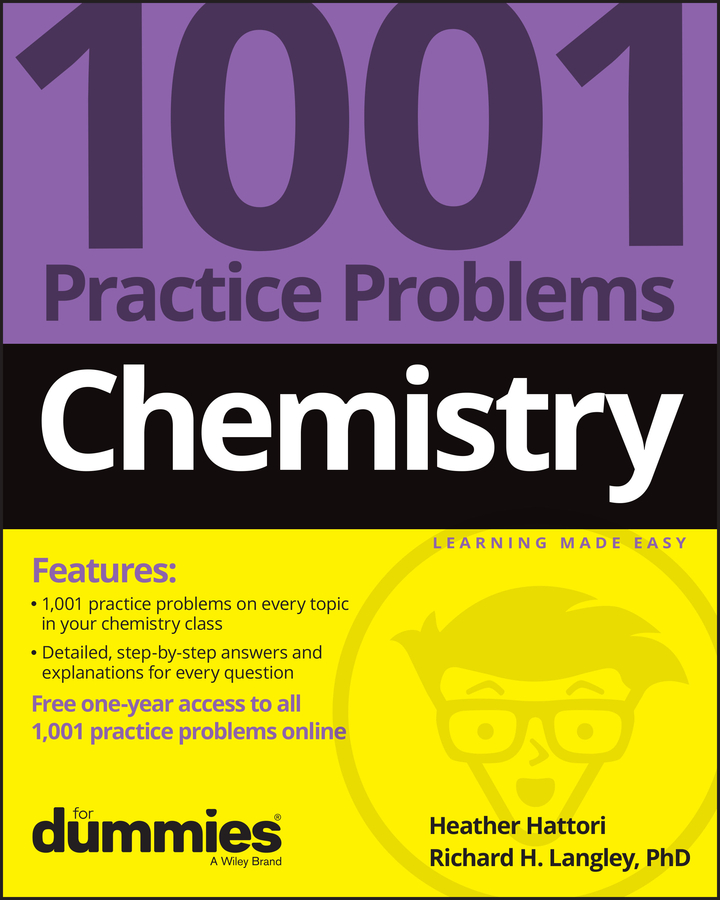You have to have a good understanding of all sorts of problems to successfully get through a chemistry class. Many of those problems require you to follow a certain process to get to the right solution: Perhaps it’s a series of rules you must follow, a conversion you need to use, or a formula you have to apply. Some of these end up being common themes in many of the chemistry problems you encounter.

In the following sections, you find some of the most common processes you need to use on a daily basis in chemistry.

## 4-question guide to naming chemistry formulas

Need to name a chemical formula and not sure where to start? Here’s a simple list that will point you in the right direction. Answer these questions as you go, and you’ll have your chemical named in no time.

1. Does the formula begin with an H?

If so, you more than likely are dealing with an acid. You must apply the rules of acid naming when dealing with these formulas. Remember, if oxygen is present, the substance is considered an oxy-acid and must be named accordingly: Find the name of the polyatomic ion that the oxy-acid contains. If the name ends in –ate, change the ending to –ic. If the name ends in –ite change the ending to –ous. Then simply write acid at the end.

If the formula contains one positive cation and one negative anion with no oxygen present, then it’s a binary acid and must be named accordingly: Write hydro, write the name of the anion present, and change the ending of the name to –ic. Then write acid after it.

2. Does the formula contain a metal (not hydrogen)?

If there’s no metal, you’re naming a covalent compound, so you need to use the covalent prefixes. Be sure to change the ending of the second element to -ide. If there is a metal, then you’re dealing with an ionic compound, so proceed to Question 3.

3. Is the cation a transition metal (Group B) or a metal with a variable charge?

If the cation is a Group B metal (or other metal of variable charge, like tin), you need to use Roman numerals to specify its charge. If the cation isn’t a transition metal and you know the charge, you don’t need to specify the charge with roman numerals.

4. Is the anion a polyatomic ion?

If so, you have to recognize it as such and have its name memorized (or easily accessible in a nifty table). If the anion isn’t a polyatomic ion, you use an -ide ending.

## A quick guide to mole conversions in chemistry

Moles are always challenging to deal with, and in a general chemistry class, you usually end up having to perform a lot of conversions involving moles (mol).

Whether you’re converting from moles to grams, moles to volume, or moles to particles (atoms or molecules), use this quick guide to remind you of how to do each type of mole conversion:

• From mass (grams) to moles: Divide your initial mass by the molar mass of the compound as determined by the periodic table.

From moles to mass (grams): Multiply your initial mole value by the molar mass of the compound as determined by the periodic table.

• From volume (liters) to moles: Divide your initial volume by the molar volume constant, 22.4L.

From moles to volume (liters): Multiply your mole value by the molar volume constant, 22.4L.

• From particles (atoms, molecules, or formula units) to moles: Divide your particle value by Avogadro’s number, 6.02 × 1023. Remember to use parentheses on your calculator!

From moles to particles (atoms, molecules, or formula units): Multiply your mole value by Avogadro’s number, 6.02 × 1023.

• Mole-to-mole conversions: Use the coefficients from your balanced equation to determine your conversion factor. Be sure your units cancel out so you end up with the correct mole value.

## How to draw Lewis electron dot structures in chemistry

Chemistry may not be much of a race, but what if you need to draw a Lewis structure as quickly as you can? Here’s a step-by-step process to make it happen, along with an example showing how you would apply these steps to draw the structure of water:

1. Write a skeletal structure showing a reasonable bonding pattern using just the element symbols.

In the case of water, H2O, oxygen is the central element and the hydrogen atoms are both bonded to it. The bonding pattern looks like this: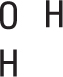2. Take all the valence electrons from all the atoms and throw them into an electron pot.

Each hydrogen atom has 1 electron, and the oxygen atom has 6 valence electrons (VIA family), so you have 8 electrons in your electron pot.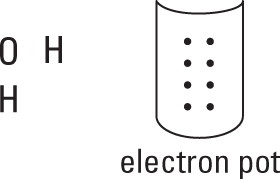3. Use the N – A = S equation to compute the number of bonds in this molecule.

In this equation,

• N equals the sum of the number of valence electrons needed by each atom.

• A is the number of valence electrons in your electron pot — the sum of the number of valence electrons available for each atom.

• S equals the number of electrons shared in the molecule; each bond involves two electrons.

So in the case of water,

• N = 8 + 2(2) = 12 (8 valence electrons for the oxygen atom, plus 2 each for the two hydrogen atoms)

• A = 6 + 2(1) = 8 (6 valence electrons for the oxygen atom, plus 1 for each of the two hydrogen atoms)

• S = 12 – 8 = 4 (four electrons shared in water), and S/2 = 4/2 = 2 bonds

4. Distribute the electrons from your electron pot to account for the bonds.

You use 4 electrons from the 8 in the pot, which leaves you with 4 to distribute later. At least one bond must connect your central atom to the atoms surrounding it.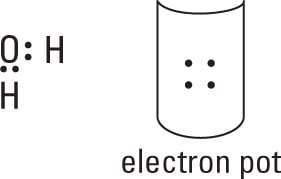5. Distribute the rest of the electrons (normally in pairs) so each atom achieves its full octet of electrons.

Here is the completed electron-dot formula for wate.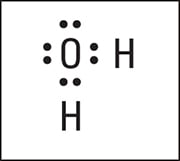Electron-dot formula of H2O.

## Pressure/temperature/volume relationships in chemistry

When you’re looking at gas laws and how pressure, temperature, and volume are related in Chemistry, remembering how they all interact with each other can be difficult.

Thankfully, there’s a very, very easy way to remember their relationships without having to remember every detail of Boyle’s law, Charles’s law, and Gay-Lussac’s law. All you need to do is remember the letters

PTV

In alphabetical order.

If you can remember that, you’ll be fine. Here’s how to interpret this three-letter helper:

• If two letters are touching each other, they have a direct relationship. That is, pressure and temperature have a direct relationship, and volume and temperature have a direct relationship. That means if one of them goes up, the other will go up, assuming the third variable is held constant. If one goes down, the other will go down.

• If two letters are not touching each other, they have an indirect relationship. That is, when pressure or volume goes up, the other will go down, assuming the other variable (temperature) is held constant.